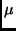Next: Learning Module Exercise Up: Output Compare Interrupts Previous: Op-amp Buffers

## How to generate a PWM signal using the MicroStamp11

The preceding section covered the hardware side of this learning module. This section covers the software side. In particular, this section shows how to use the OC4 interrupt to generate a pulse width modulated signal.

Recall that the OC4han() ISR is called every time the output compare register TOC4 equals the counter register TCNT. Also recall that the generation of the output compare event can also change the state of an output pin if the appropriate bit in the control register TCTL1 is set. We can use these two facts to revise the OC4han() function so it automatically generates a pulse width modulated wave.

The following listing shows the init() function and the revised OC4han() function.

 unsigned int _Time;
unsigned int _rate_high;
unsigned int _rate_low;

#pragma interrupt_handler OC4han()
void OC4han(void){
if(TCTL1 == 0x08){
TOC4 = TOC4 + _rate_high;
TCTL1 |= OM4; TCTL1 |= OL4;
_Time=_Time+1;
}else{
TOC4 = TOC4 + _rate_low;
TCTL1 |= OM4; TCTL1 &= ~OL4;
}
TFLG1 |= OC4F;
}

void init(void){
asm(" sei");
CONFIG = 0x04;
_Time=0;
_rate_low = 127;
_rate_high = 128;
TMSK2 = 0x03;
TMSK1 |= OC4I;
TFLG1 |= OC4F;
TCTL1 = OM4;
TOC4 = TCNT+_rate_low;

asm(" cli");
}


Let's first look at the global variables associated with our revised OC4 handler. There is the usual _Time global variable that we used earlier. But we have also introduced two additional global variables _rate_low and _rate_high. These two global variables are used to set the duty cycle of the pwm signal.

Now let's look at the initialization function init(). This function initializes _Time to zero and initializes the variables _rate_low and _rate_high to 127 and 128, respectively. The instruction TMSK2 = 0x03 sets the clock update rate at 500 nanoseconds. The instruction TMSK1 |= OC4I enables the OC4 interrupt and the instruction TFLG1 |= OC4F acknowledges any previously caught OC4 interrupts. The next instruction is  TCTL1 = OM4. OM4 is the logical name for one of the bits in the TCTL1 register. When this bit is set, then the output pin OC4 is cleared (set to zero). The final instruction in the initialization function (TOC4 = TCNT+_rate_low) sets the deadline for the next OC4 event.

What our initialization function has done is to set the output pin OC4 low. The next OC4 event is triggered _rate_low ticks into the future. When this occurs then the OC4han() is executed. So now let's look at the revised OC4han() function.

The first thing we note here is that our handler is much more complex than the original OC4han() function we used before. In this case, the first thing the ISR does is check to see if TCTL1 == OM4. If this happens, then we know that the output pin OC4 is low. So we reset the output compare register TOC4 to trigger _rate_high ticks into the future and then set the output pin high using the instructions TCTL1 |= OM4 and TCTL1 |= OL4. We then have the global time variable _Time.

If TCTL does not equal OM4, then we know that this OC4 interrupt must have occurred when the output pin OC4 was high. We then set the output pin low with the instructions TCTL1 |= OM4 and TCTL1 &= ~OL4. The output compare register TOC4 is then set to trigger the output compare event _rate_high ticks into the future.

There are a couple of things to note about this function. First, we see that it forces the output pin OC4 to be toggled between its high and low state. The amount of time that the pin remains low is given by the global variable _rate_low. The amount of time that the pin remains high is given by the global variable _rate_high. This change in pin state occurs every time we execute the OC4han function. The end result is that the voltage over pin OC4 is a pulse width modulated wave whose duty cycle is given by

duty_cycle = _rate_high/(_rate_high + _rate_low);

Initially _rate_high and _rate_low are set to 128 and 127, respectively. So when our program starts the output pin OC4 is already generating a 50 percent duty cycle PWM signal.

Note that the variables _rate_high and _rate_low are both global. This means that they can be set from within your program to modify the duty cycle of the PWM signal on pin OC4. A simple function that modifies this duty cycle is given below.

  void set_pwm(short idur){
short ilow = 20;
short ihigh = 240;
if( idur >= ihigh) idur=ihigh;
if( idur <= ilow) idur=ilow;
_rate_high = idur;
_rate_low = 255-idur;
}

All that this function does is set the global variables _rate_high and _rate_low. The period of the PWM wave is set to 256 ticks or 128seconds. The duty cycle is determined by the input variable idur. Note that we've provided some clipping on the variable idur in order to keep it in the range between 20 and 240. The reason for this is that if we go outside of these ranges, then the shorter half of the PWM wave is so short that the OC4 interrupt will fire before the interrupt handler has completed executing. This type of timing problem can often be very difficult to debug.Next: Learning Module Exercise Up: Output Compare Interrupts Previous: Op-amp Buffers
Bill Goodwine 2002-09-29